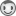## How do I vectorize a DO loop - static markov chain (SAS/IML)

I am new to vectorizing generally and SAS/IML though have quickly realised the potential program and computational savings.  I have a specific problem I have set out below and have searched the internet, to no avail.  I have been inspired by the solution to another problem in this link - in which a series calculation is vectorized from a DO loop and read a lot of Rick Wicklin's blog which have helped massively.

Problem: I have a transition matrix (e.g. 4x4) and I want to forecast the volume movement between the 4 states over N months. The final result will be a Nx4 matrix with the forecasted volumes in each state.  I can achieve this using a DO loop but I am hopeful that someone can help me find a vectorized solution, as I may have to run similar vectorize this problem.

The attached files contains an example of my current approach (using a DO loop).

Andrew

1 ACCEPTED SOLUTION

Accepted Solutions

## Re: How do I vectorize a DO loop - static markov chain (SAS/IML)

This is an iterative computation, so you can't avoid the DO loop. If you are only interested in the asymptotic steady-state solution, there are ways to find that directly by using eigenvalues, but if you want the forecast time series then you have to compute each step.

There is a way to make your program more efficient. Currently you are computing a matrix power (trans**i), which is an expensive computation at each stepo of the loop. At the i_th step you are repeating all the computations from the previous step, plus one more matrix multiplication.

However, you can reduce this to N matrix-vector computations, which is much faster.  Inside the loop, merely update the previous computation, like this:

``````v = input1;
do i = 1 to ForecastMonths ;
v = v * trans;
results[i, ] = v;
end ;
``````

It isn't clear whether you want the first row to be the 0th iteration or the result after the first iteration. I've done the latter.

Not only is this method faster, but it is numerically more stable because you never form large powers of matrices.

7 REPLIES 7

## Re: How do I vectorize a DO loop - static markov chain (SAS/IML)

This is an iterative computation, so you can't avoid the DO loop. If you are only interested in the asymptotic steady-state solution, there are ways to find that directly by using eigenvalues, but if you want the forecast time series then you have to compute each step.

There is a way to make your program more efficient. Currently you are computing a matrix power (trans**i), which is an expensive computation at each stepo of the loop. At the i_th step you are repeating all the computations from the previous step, plus one more matrix multiplication.

However, you can reduce this to N matrix-vector computations, which is much faster.  Inside the loop, merely update the previous computation, like this:

``````v = input1;
do i = 1 to ForecastMonths ;
v = v * trans;
results[i, ] = v;
end ;
``````

It isn't clear whether you want the first row to be the 0th iteration or the result after the first iteration. I've done the latter.

Not only is this method faster, but it is numerically more stable because you never form large powers of matrices.

## Re: How do I vectorize a DO loop - static markov chain (SAS/IML)

Thank you very much for the prompt response.  Reading your (and other) other blog entries I was perhaps being overly hopeful there was a way to vectorize everything in my life, so it is helpful to get confirmation where a loop is required.

Also, thank you for the advice regarding the vector/matrix multiplication, as opposed to the matrix-power operation.Andrew

## Re: How do I vectorize a DO loop - static markov chain (SAS/IML)

A good rule of thumb is to look for a vectorized approach when your loop is over the rows or columns of a matrix or vector.

In this case, the loop is an independent of the size of the matrix.

Another possible opportunity for vectorization is when you are performin an operation on a VECTOR within a loop, and the (i+1)st computation is INDEPENDENT of the result of the i_th computation.  Then you might be able to pack all those vectors into a matrix and do one columnwise or rowwise operation on the matrix.  Alas, that is not the case here: the (i+1)st computation depends on the result of the i_th computation.

## Re: How do I vectorize a DO loop - static markov chain (SAS/IML)

Thanks Rick.

I understood from your other blog posts that the dependency of the (i+1)th observation on the ith means that vectorization wouldn't be possible. However, it was when someone showed me that I can get to the ith iteration by raising the transition matrix to the ith power that I thought there might be a way to do this in one step as I only require the initial vector and the transition matrix**i.  However, I note your point about matrix power being more expensive than matrix multiplication.

That is disappointing.  However, I'm on a mission as I'm working with some heavily looped code at the moment and there are some definite wins for me.

Cheers,

Andrew

## Re: How do I vectorize a DO loop - static markov chain (SAS/IML)

I wrote down some of my thoughts on how to iterate a Markov transition matrix efficiently in SAS/IML. See

Markov transition matrices in SAS/IML

## Re: How do I vectorize a DO loop - static markov chain (SAS/IML)

For future reference, I wrote up some of these ideas and related concepts for analyzing Markov chains:

Markov Transition Matrices in SAS/IML

Absorbing Markov Chains in SAS

## Re: How do I vectorize a DO loop - static markov chain (SAS/IML)

Thanks for sharing these Rick. I have been following your blog with interest!
From The DO Loop